## Ellipse

An ellipse is the set of points in a plane such that the sum of the distances from two fixed points in that plane stays constant. The two points are each called a focus. The plural of focus is foci. The midpoint of the segment joining the foci is called the center of the ellipse. An ellipse has two axes of symmetry. The longer one is called the major axis, and the shorter one is called the minor axis. The two axes intersect at the center of the ellipse (see Figure 1).

Figure 1. Axes and foci of ellipses.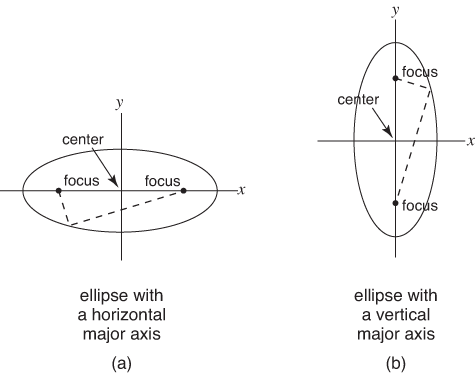The equation of an ellipse that is centered at (0, 0) and has its major axis along the x‐axis has the following standard form.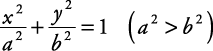The length of the major axis is 2| a|, and the length of the minor axis is 2| b|. The endpoints of the major axis are ( a, 0) and (– a, 0) and are referred to as the major intercepts. The endpoints of the minor axis are (0, b) and (0, – b) and are referred to as the minor intercepts. If ( c, 0) and (– c, 0) are the locations of the foci, then c can be found using the equation

c 2 = a 2b 2

If an ellipse has its major axis along the y‐axis and is centered at (0, 0), the standard form becomes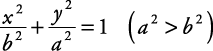The endpoints of the major axis become (0, a) and (0, – a). The endpoints of the minor axis become ( b, 0) and (– b, 0). The foci are at (0, c) and (0, – c), with

c 2 = a 2b 2

When an ellipse is written in standard form, the major axis direction is determined by noting which variable has the larger denominator. The major axis either lies along that variable's axis or is parallel to that variable's axis.

##### Example 1

Graph the following ellipse. Find its major intercepts, length of the major axis, minor intercepts, length of the minor axis, and foci.This ellipse is centered at (0, 0). Since the larger denominator is with the y variable, the major axis lies along the y‐axis.Major intercepts: (0, 3), (0, –3)

Length of major axis: 2 | a| = 6

Minor intercepts: (2, 0), (–2, 0)

Length of minor axis: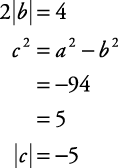Foci: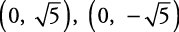The graph of this ellipse is shown in Figure 2.